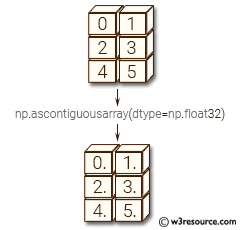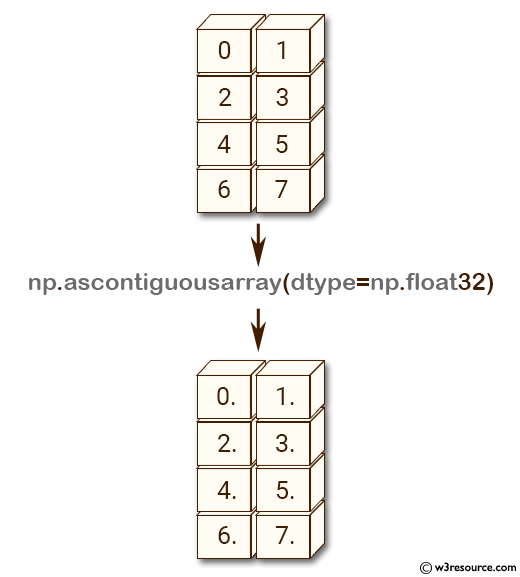# NumPy: numpy.ascontiguousarray() function

## numpy.ascontiguousarray() function

The numpy.ascontiguousarray() function is used to get a contiguous array in memory (C order). np.ascontiguousarray() function is useful when working with arrays that have a non-contiguous memory layout, as it can improve performance by ensuring that the data is stored in contiguous memory locations.

Syntax:

`numpy.ascontiguousarray(a, dtype=None)`Parameters:

Name Description Required /
Optional
a Input array. Required
dtype Data-type of returned array. optional

Return value:

Contiguous array of same shape and content as a, with type dtype if specified.

Example: Creating a contiguous copy of an array with a specified data type

``````>>> import numpy as np
>>> a = np.arange(8). reshape (4, 2)
>>> np.ascontiguousarray(a, dtype=np.float32)
array([[ 0.,  1.],
[ 2.,  3.],
[ 4.,  5.],
[ 6.,  7.]], dtype=float32)
``````

In the above example the second line of code creates a 2-dimensional numpy array a with shape (4, 2) using np.arange() function and reshaping the output to the specified shape.

Then np.ascontiguousarray() function is applied on 'a' with the specified data type np.float32. This function returns a contiguous array, which may be a new view of the original array if the original array was already contiguous with the same data type, or a new copy of the original array with the desired data type.

Pictorial Presentation:Example: Contiguous memory layout using np.ascontiguousarray()

``````>>> import numpy as np
>>> a = np.arange(8). reshape (4, 2)
>>> np.ascontiguousarray(a, dtype=np.float32)
array([[ 0.,  1.],
[ 2.,  3.],
[ 4.,  5.],
[ 6.,  7.]], dtype=float32)
>>> a.flags['C_CONTIGUOUS']
True
``````

In the above code, a two-dimensional array a is created using the arange() and reshape() functions. Then, the np.ascontiguousarray() function is used to create a new array that has a contiguous memory layout. The dtype parameter is used to specify that the new array should have a data type of np.float32.

Python - NumPy Code Editor:

Previous: asanyarray()
Next: asmatrix()

﻿